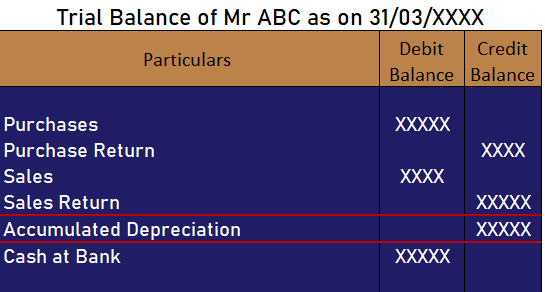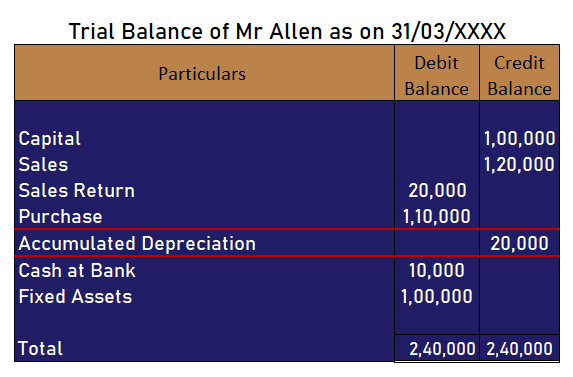# How is accumulated depreciation shown in trial balance?

-This question was submitted by a user and answered by a volunteer of our choice.

## Accumulated Depreciation in a Trial Balance

The accumulated depreciation is shown as a “credit item” in the trial balance. Accumulated depreciation is nothing but the sum total of depreciation charged until a specified date. Since in every reporting period, a part of a fixed asset is written off i.e depreciated such accumulated depreciation has a credit balance.

You should have a glance at the image of an extract of the trial balance given- below it will definitely answer your question in a more effective way.## Accumulated Depreciation

As mentioned earlier the accumulated depreciation is the sum total of depreciation that an entity has expensed in its profit and loss statement till that date. It’s basically a contra asset account as it reduces the balance in the asset account.

Illustrative Example,

Prepare a trial balance of Mr Allen on the basis of given heads of accounts;

 Particulars Amount Capital 1,00,000 Sales 1,20,000 Purchases 1,10,000 Sales Return 20,000 Fixed Assets 1,00,000 Cash at bank 10,000 Accumulated Depreciation 20,000

Solution :###### Subscribe
* indicates required